#### 一:hashMap底层实现原理和常见的面试题

2020-04-28 2374 ℃

# 基础

### 概述

HashMap基于Map接口实现，元素以键值对的方式存储，并且允许使用null键和null值,因为key不允许重复，因此只能有一个键为null,另外HashMap不能保证放入元素的顺序，它是无序的，和放入的顺序并不能相同。HashMap是线程不安全的。

### 继承关系

``````public class HashMap<K,V>extends AbstractMap<K,V>
implements Map<K,V>, Cloneable, Serializable
``````

### 基本属性

``````
static final int DEFAULT_INITIAL_CAPACITY = 1 << 4; //默认初始化大小 16
static final float DEFAULT_LOAD_FACTOR = 0.75f;     //负载因子0.75
static final Entry<?,?>[] EMPTY_TABLE = {};         //初始化的默认数组
transient int size;     //HashMap中元素的数量
int threshold;          //判断是否需要调整HashMap的容量
``````

Note：HashMap的扩容操作是一项很耗时的任务，所以如果能估算Map的容量，最好给它一个默认初始值，避免进行多次扩容。HashMap的线程是不安全的，多线程环境中推荐是ConcurrentHashMap。

### 常被问到的HashMap和Hashtable的区别

#### 1.线程安全

Hashtable的实现方法里面都添加了synchronized关键字来确保线程同步，因此相对而言HashMap性能会高一些，我们平时使用时若无特殊需求建议使用HashMap，在多线程环境下若使用HashMap需要使用Collections.synchronizedMap()方法来获取一个线程安全的集合。

Note: Collections.synchronizedMap()实现原理是Collections定义了一个SynchronizedMap的内部类，这个类实现了Map接口，在调用方法时使用synchronized来保证线程同步,当然了实际上操作的还是我们传入的HashMap实例，简单的说就是Collections.synchronizedMap()方法帮我们在操作HashMap时自动添加了synchronized来实现线程同步，类似的其它Collections.synchronizedXX方法也是类似原理。

#### 针对null的不同

HashMap可以使用null作为key，而Hashtable则不允许null作为key

Note：HashMap以null作为key时，总是存储在table数组的第一个节点上。

#### 继承结构

HashMap是对Map接口的实现，HashTable实现了Map接口和Dictionary抽象类。

#### 初始容量与扩容

HashMap的初始容量为16，Hashtable初始容量为11，两者的填充因子默认都是0.75。

HashMap扩容时是当前容量翻倍即:capacity2，Hashtable扩容时是容量翻倍+1即:capacity2+1。

#### 两者计算hash的方法不同

``````int hash = key.hashCode();
int index = (hash & 0x7FFFFFFF) % tab.length;
``````

HashMap计算hash对key的hashcode进行了二次hash，以获得更好的散列值，然后对table数组长度取摸。

``````int hash = hash(key.hashCode());
int i = indexFor(hash, table.length);

static int hash(int h) {
// This function ensures that hashCodes that differ only by
// constant multiples at each bit position have a bounded
// number of collisions (approximately 8 at default load factor).
h ^= (h >>> 20) ^ (h >>> 12);
return h ^ (h >>> 7) ^ (h >>> 4);
}

static int indexFor(int h, int length) {
return h & (length-1);

``````

# 底层实现原理

### 哈希表结构的优势？

``````哈希表作为一种优秀数据结构

``````

### 哈希算法？

``````h 通过hash算法计算得到的的一个整型数值
h可以近似看做一个由key的hashcode生成的随机数，区别在于相同的hashcode生成的h必然相同

(ps:hash碰撞只能尽量避免，而无法杜绝,由于h是一个固定长度整型数据,原则上只要有足够多的输入，就一定会产生碰撞)

``````

### JDK1.8中对HashMap做了哪些改动？

• 默认初始化容量=0
• 引入红黑树，优化数据结构
• 将链表头插法改为尾插法，解决1.7中多线程循环链表的bug
• 优化hash算法
• resize计算索引位置的算法改进
• 先插入后扩容

### JDK1.8后的储存结构

HashMap由数组和链表来实现对数据的存储
HashMap采用Entry数组来存储key-value对，每一个键值对组成了一个Entry实体，Entry类实际上是一个单向的链表结构，它具有Next指针，可以连接下一个Entry实体，以此来解决Hash冲突的问题。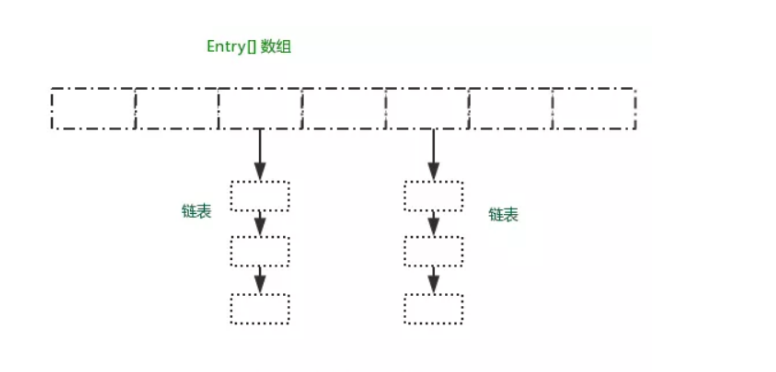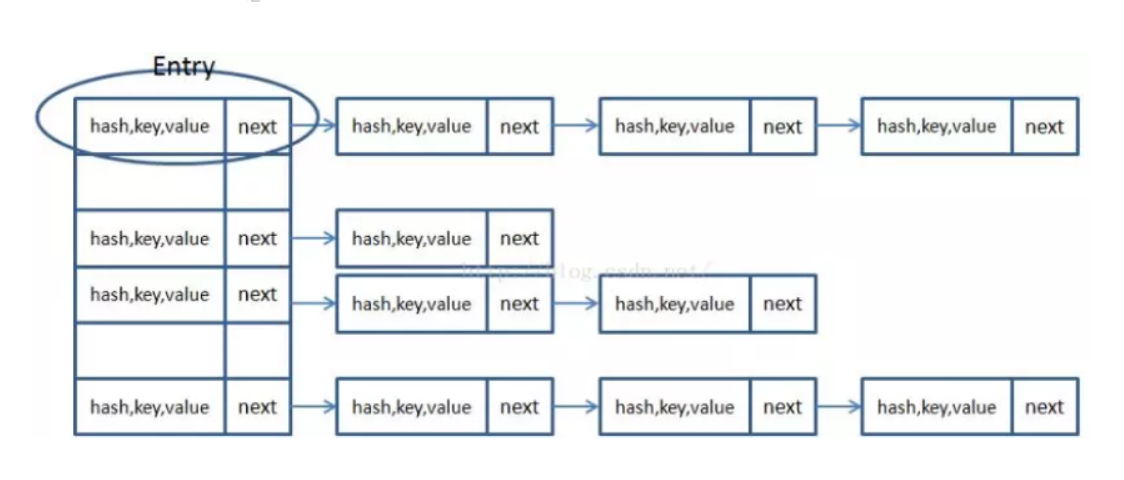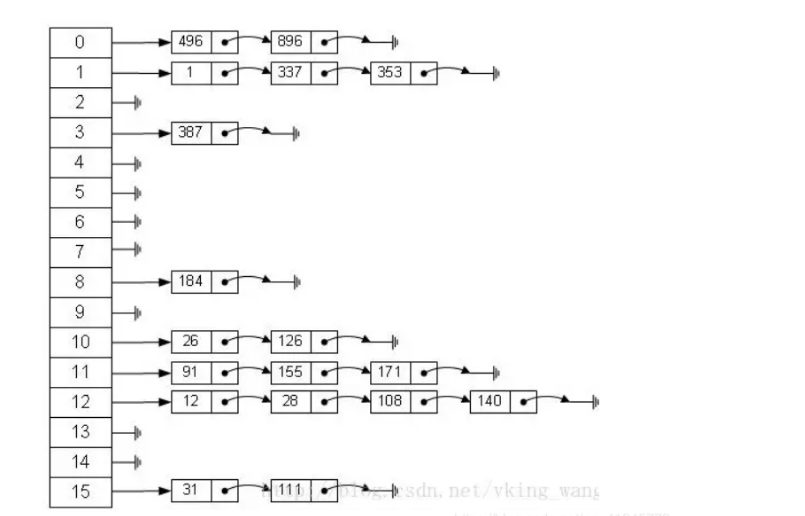HashMap里面实现一个静态内部类Entry，其重要的属性有 hash，key，value，next。

HashMap里面用到链式数据结构的一个概念。上面我们提到过Entry类里面有一个next属性，作用是指向下一个Entry。打个比方， 第一个键值对A进来，通过计算其key的hash得到的index=0，记做:Entry = A。一会后又进来一个键值对B，通过计算其index也等于0，现在怎么办？HashMap会这样做:B.next = A,Entry = B,如果又进来C,index也等于0,那么C.next = B,Entry = C；这样我们发现index=0的地方其实存取了A,B,C三个键值对,他们通过next这个属性链接在一起。所以疑问不用担心。也就是说数组中存储的是最后插入的元素。到这里为止，HashMap的大致实现，我们应该已经清楚了

#### JDK1.8之后的变化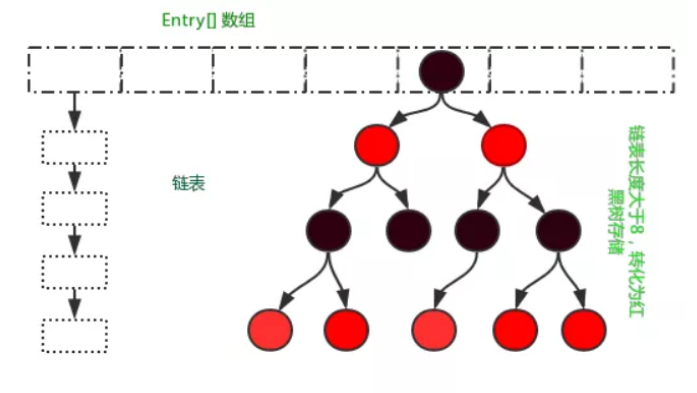### HashMap中put()的过程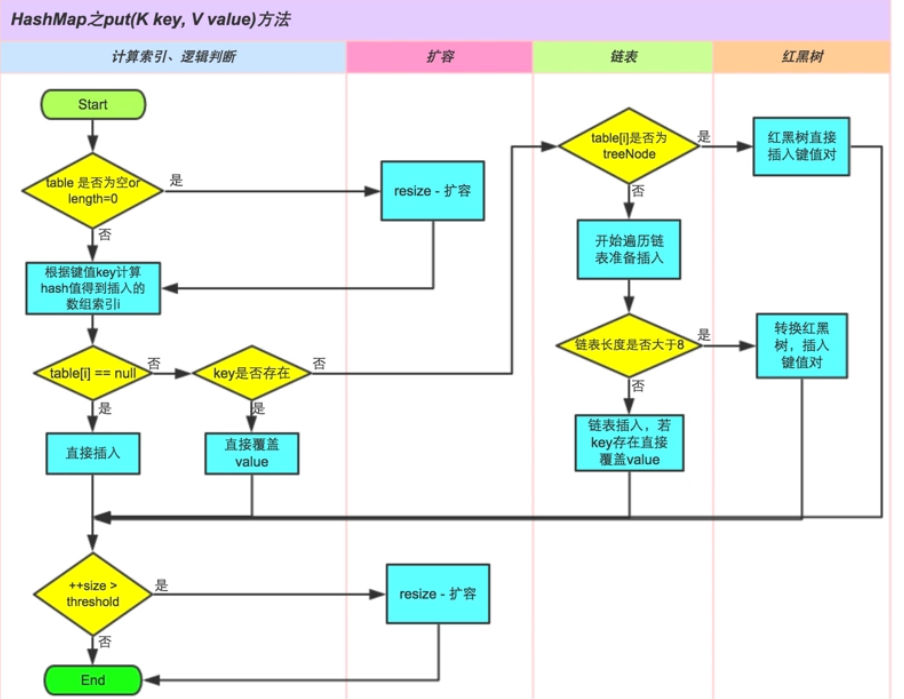``````public V put(K key, V value) {
return putVal(hash(key), key, value, false, true);
}
final V putVal(int hash, K key, V value, boolean onlyIfAbsent,
boolean evict) {
Node<K,V>[] tab; Node<K,V> p; int n, i;
// 判断数组是否为空，长度是否为0，是则进行扩容数组初始化
if ((tab = table) == null || (n = tab.length) == 0)
n = (tab = resize()).length;
// 通过hash算法找到数组下标得到数组元素，为空则新建
if ((p = tab[i = (n - 1) & hash]) == null)
tab[i] = newNode(hash, key, value, null);
else {
Node<K,V> e; K k;
// 找到数组元素，hash相等同时key相等，则直接覆盖
if (p.hash == hash &&
((k = p.key) == key || (key != null && key.equals(k))))
e = p;
// 该数组元素在链表长度>8后形成红黑树结构的对象,p为树结构已存在的对象
else if (p instanceof TreeNode)
e = ((TreeNode<K,V>)p).putTreeVal(this, tab, hash, key, value);
else {
// 该数组元素hash相等，key不等，同时链表长度<8.进行遍历寻找元素，有就覆盖无则新建
for (int binCount = 0; ; ++binCount) {
if ((e = p.next) == null) {
// 新建链表中数据元素，尾插法
p.next = newNode(hash, key, value, null);
if (binCount >= TREEIFY_THRESHOLD - 1) // -1 for 1st
// 链表长度>=8 结构转为 红黑树
treeifyBin(tab, hash);
break;
}
if (e.hash == hash &&
((k = e.key) == key || (key != null && key.equals(k))))
break;
p = e;
}
}
// 新值覆盖旧值
if (e != null) { // existing mapping for key
V oldValue = e.value;
// onlyIfAbsent默认false
if (!onlyIfAbsent || oldValue == null)
e.value = value;
afterNodeAccess(e);
return oldValue;
}
}
++modCount;
// 判断是否需要扩容
if (++size > threshold)
resize();
afterNodeInsertion(evict);
return null;
}
``````

• 1.检查数组是否为空，执行resize()扩充；在实例化HashMap时，并不会进行初始化数组）

• 2.通过hash值计算数组索引，获取该索引位的首节点。

• 3.如果首节点为null（没发生碰撞），则创建新的数组元素，直接添加节点到该索引位(bucket)。

• 4.如果首节点不为null（发生碰撞），那么有3种情况

• ① key和首节点的key相同，覆盖old value（保证key的唯一性）；否则执行②或③

• ② 如果首节点是红黑树节点（TreeNode），将键值对添加到红黑树。

• ③ 如果首节点是链表，进行遍历寻找元素，有就覆盖无则新建，将键值对添加到链表。添加之后会判断链表长度是否到达TREEIFY_THRESHOLD - 1这个阈值，“尝试”将链表转换成红黑树。

• 5.最后判断当前元素个数是否大于threshold，扩充数组。

### HashMap中get()过程

``````public V get(Object key) {
Node<K,V> e;
return (e = getNode(hash(key), key)) == null ? null : e.value;
}

final Node<K,V> getNode(int hash, Object key) {
Node<K,V>[] tab; Node<K,V> first, e; int n; K k;
if ((tab = table) != null && (n = tab.length) > 0 &&
(first = tab[(n - 1) & hash]) != null) {
// 永远检查第一个node
if (first.hash == hash && // always check first node
((k = first.key) == key || (key != null && key.equals(k))))
return first;
if ((e = first.next) != null) {
if (first instanceof TreeNode)  // 树查找
return ((TreeNode<K,V>)first).getTreeNode(hash, key);
do {
if (e.hash == hash &&   // 遍历链表
((k = e.key) == key || (key != null && key.equals(k))))
return e;
} while ((e = e.next) != null);
}
}
return null;
}
``````

• 1.根据key计算hash;
• 2.检查数组是否为空，为空返回null;
• 3.根据hash计算bucket位置，如果bucket第一个元素是目标元素，直接返回。否则执行4;
• 4.如果bucket上元素大于1并且是树结构，则执行树查找。否则执行5;
• 5.如果是链表结构，则遍历寻找目标

### HashMap中resize()过程

`````` final Node<K,V>[] resize() {
Node<K,V>[] oldTab = table;
int oldCap = (oldTab == null) ? 0 : oldTab.length;
int oldThr = threshold;
int newCap, newThr = 0;
if (oldCap > 0) {
// 如果已达到最大容量不在扩容
if (oldCap >= MAXIMUM_CAPACITY) {
threshold = Integer.MAX_VALUE;
return oldTab;
}
// 通过位运算扩容到原来的两倍
else if ((newCap = oldCap << 1) < MAXIMUM_CAPACITY &&
oldCap >= DEFAULT_INITIAL_CAPACITY)
newThr = oldThr << 1; // double threshold
}
else if (oldThr > 0) // initial capacity was placed in threshold
newCap = oldThr;
else {               // zero initial threshold signifies using defaults
newCap = DEFAULT_INITIAL_CAPACITY;
}
if (newThr == 0) {
float ft = (float)newCap * loadFactor;
newThr = (newCap < MAXIMUM_CAPACITY && ft < (float)MAXIMUM_CAPACITY ?
(int)ft : Integer.MAX_VALUE);
}
// 新的扩容临界值
threshold = newThr;
@SuppressWarnings({"rawtypes","unchecked"})
Node<K,V>[] newTab = (Node<K,V>[])new Node[newCap];
table = newTab;
if (oldTab != null) {
for (int j = 0; j < oldCap; ++j) {
Node<K,V> e;
if ((e = oldTab[j]) != null) {
oldTab[j] = null;
// 如果该位置元素没有next节点，将该元素放入新数组
if (e.next == null)
newTab[e.hash & (newCap - 1)] = e;
else if (e instanceof TreeNode)
// 树节点
((TreeNode<K,V>)e).split(this, newTab, j, oldCap);
else { // preserve order
// 链表节点。

// lo串的新索引位置与原先相同
Node<K,V> loHead = null, loTail = null;
// hi串的新索引位置为[原先位置j+oldCap]
Node<K,V> hiHead = null, hiTail = null;
Node<K,V> next;
do {
next = e.next;
// 原索引，oldCap是2的n次方，二进制表示只有一个1，其余是0
if ((e.hash & oldCap) == 0) {
if (loTail == null)
else
// 尾插法
loTail.next = e;
loTail = e;
}
// 原索引+oldCap
else {
if (hiTail == null)
else
hiTail.next = e;
hiTail = e;
}
} while ((e = next) != null);
// 根据hash判断该bucket上的整个链表的index还是旧数组的index，还是index+oldCap
if (loTail != null) {
loTail.next = null;
}
if (hiTail != null) {
hiTail.next = null;
}
}
}
}
}
return newTab;
}
``````

JDK1.8版本中扩容相对复杂。在1.7版本中，重新根据hash计算索引位置即可；而在1.8版本中分2种情况，下边用图例来解释。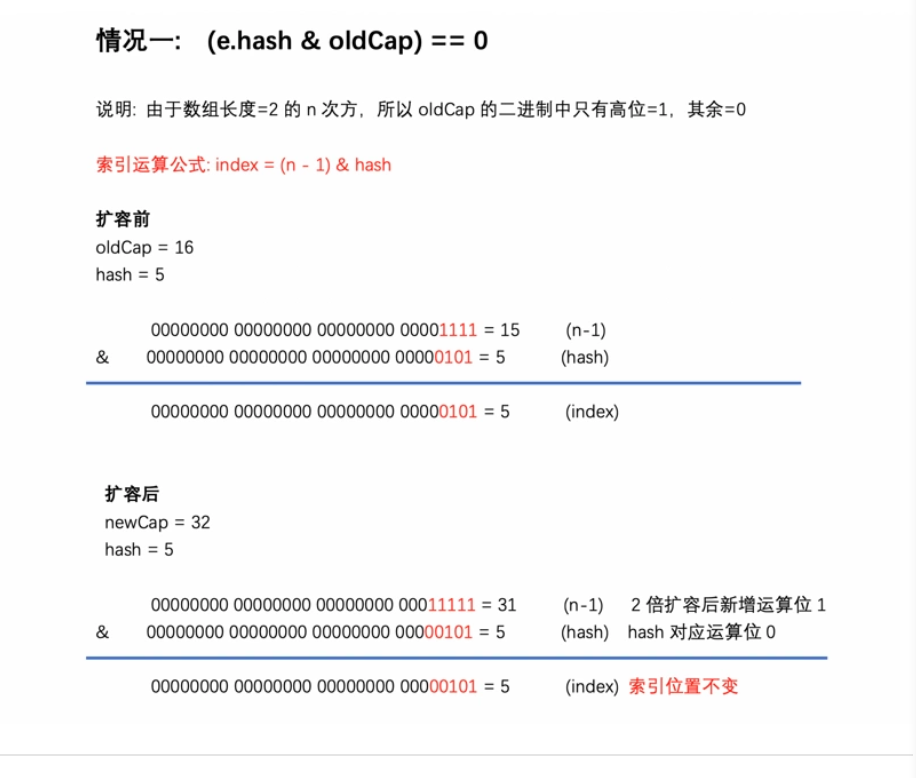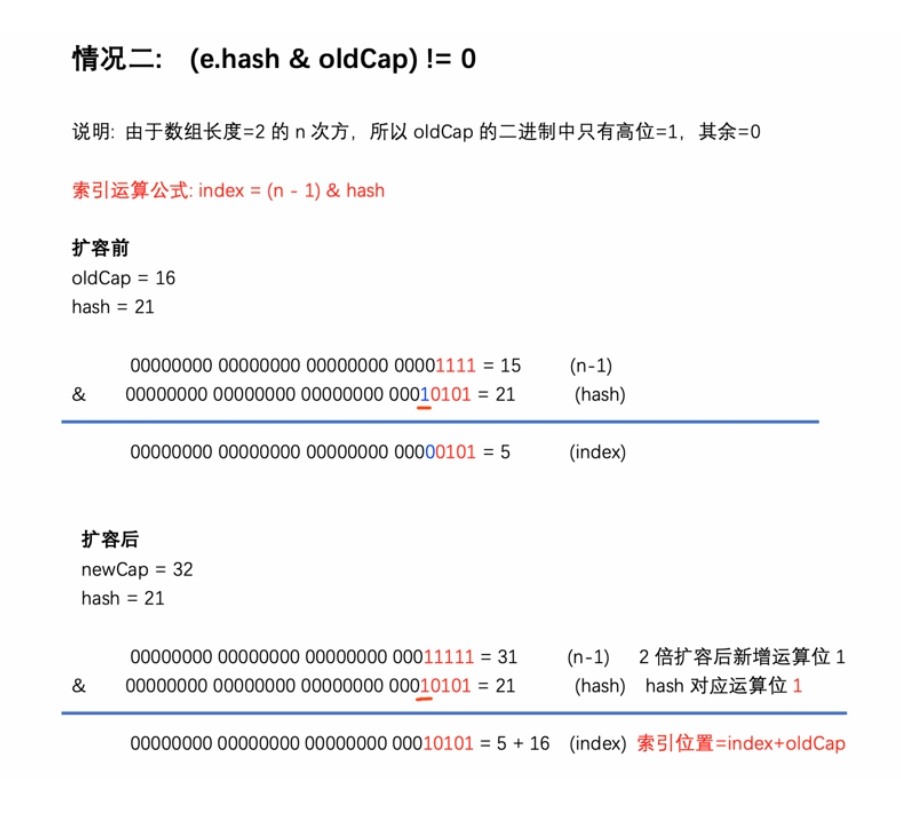https://segmentfault.com/a/1190000021928659